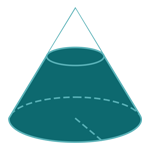Report a Problem
Suggestions

#Calculate The Surface Area of A Truncated Cone/Conical Frustum

Last updated: Saturday, June 24, 2023
Select a type of cone below
Right Cone
Truncated Cone

The surface area of a truncated cone or conical frustum can be understood as the sum of the areas of its individual faces.

To find the surface area, we need to find the area of the top and bottom circular faces, as well as the lateral surface area. The top and bottom faces are simply circles, so their areas can be found using the formula for the area of a circle.

The lateral surface area of a truncated cone is a bit more complex. It is essentially the area of the curved surface that makes up the sides of the frustum. This can be found by first calculating the slant height of the frustum using the Pythagorean theorem, and then using that to find the length of the generatrix (the slant height projected onto the lateral surface). Finally, we can use the length of the generatrix and the perimeter of the circle at the bottom of the frustum to calculate the lateral surface area.

Real-life examples of truncated cones can be found in various places, such as lampshades, storage tanks, and the shape of certain buildings. For example, the iconic shape of the Transamerica Pyramid in San Francisco is that of a tapered pyramid or frustum. The surface area of the frustum was a key consideration in its construction and engineering.

The formula for determining the surface area of a truncated cone/conical frustum is defined as:
$$SA$$ $$=$$ $$\pi$$ $$\cdot$$ $$(R$$ $$+$$ $$r)$$ $$\cdot$$ $$l$$ $$+$$ $$\pi$$ $$\cdot$$ $$(R^2$$ $$+$$ $$r^2)$$
$$SA$$: the surface area of the truncated cone/conical frustum
$$r$$: the radius of the top
$$R$$: the radius of the base
$$l$$: the length of the slant height
The SI unit of surface area is: $$square \text{ } meter\text{ }(m^2)$$
Select a problem below
Find $$SA$$
Find $$SA$$

## Find $$SA$$

Use this calculator to determine the surface area of a truncated cone when the slant height and the radii of both the top and base are given.
Hold & Drag
CLOSE
$$r$$
$$meter$$
$$R$$
$$meter$$
$$l$$
$$meter$$